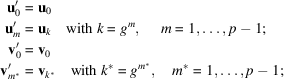International
Tables for
Crystallography
Volume B
Reciprocal space
Edited by U. Shmueli

International Tables for Crystallography (2010). Vol. B, ch. 1.3, pp. 55-56   | 1 | 2 |

Section 1.3.3.2.3.1. N an odd prime

G. Bricognea

aGlobal Phasing Ltd, Sheraton House, Suites 14–16, Castle Park, Cambridge CB3 0AX, England, and LURE, Bâtiment 209D, Université Paris-Sud, 91405 Orsay, France

1.3.3.2.3.1. N an odd prime

| top | pdf |

The ringhas the property that itsnonzero elements, called units, form a multiplicative group. In particular, all unitshave a unique multiplicative inverse in, i.e. a unitsuch that. This endowswith the structure of a finite field.

Furthermore,is a cyclic group, i.e. consists of the successive powersof a generator g called a primitive root mod p (such a g may not be unique, but it always exists). For instance, for,is generated by, whose successive powers mod 7 are:[see Apostol (1976), Chapter 10].

The basis of Rader's algorithm is to bring to light a hidden regularity in the matrixby permuting the basis vectorsandofas follows:where g is a primitive root mod p.

With respect to these new bases, the matrix representingwill have the following elements:Thus the `core'of matrix, of size, formed by the elements with two nonzero indices, has a so-called skew-circulant structure because elementdepends only on. Simplification may now occur because multiplication byis closely related to a cyclic convolution. Introducing the notationwe may write the relationin the permuted bases aswhere Z is defined by,.

Thusmay be obtained by cyclic convolution of C and Z, which may for instance be calculated bywhere × denotes the component-wise multiplication of vectors. Since p is odd,is always divisible by 2 and may even be highly composite. In that case, factoringby means of the Cooley–Tukey or Good methods leads to an algorithm of complexity p log p rather thanfor. An added bonus is that, because, the elements ofcan be shown to be either purely real or purely imaginary, which halves the number of real multiplications involved.

References

Apostol, T. M. (1976). Introduction to Analytic Number Theory. New York: Springer-Verlag.